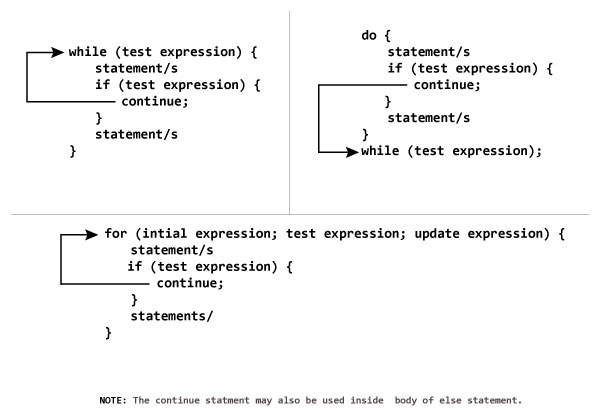## Search

### 3. C++ while and do...while Loop

Loops are used in programming to repeat a specific block of code. In this article, you will learn to create while and do...while loops in C++ programming.

In computer programming, loop repeats a certain block of code until some end condition is met.
There are 3 type of loops in C++ Programming:

## C++ while Loop

The syntax of a while loop is:
```while (testExpression)
{
// codes
}```

### How while loop works?

• The while loop evaluates the test expression.
• If the test expression is true, codes inside the body of while loop is evaluated.
• Then, the test expression is evaluated again. This process goes on until the test expression is false.
• When the test expression is false, while loop is terminated.

### Example 1: C++ while Loop

``````// C++ Program to compute factorial of a number
// Factorial of n = 1*2*3...*n

#include <iostream>
using namespace std;

int main()
{
int number, i = 1, factorial = 1;

cout << "Enter a positive integer: ";
cin >> number;

while ( i <= number) {
factorial *= i;      //factorial = factorial * i;
++i;
}

cout<<"Factorial of "<< number <<" = "<< factorial;
return 0;
}``````
Output
```Enter a positive integer: 4
Factorial of 4 = 24```
In this program, user is asked to enter a positive integer which is stored in variable number. Let's suppose, user entered 4.

Then, the while loop starts executing the code. Here's how while loop works:
1. Initially, `i = 1`, test expression `i <= number` is true and factorial becomes 1.
2. Variable i is updated to 2, test expression is `true`, factorial becomes 2.
3. Variable i is updated to 3, test expression is `true`, factorial becomes 6.
4. Variable i is updated to 4, test expression is `true`, factorial becomes 24.
5. Variable i is updated to 5, test expression is `false` and while loop is terminated.

## C++ do...while Loop

The do...while loop is a variant of the while loop with one important difference. The body of do...while loop is executed once before the test expression is checked.
The syntax of do..while loop is:
```do {
// codes;
}
while (testExpression);```

### How do...while loop works?

• The codes inside the body of loop is executed at least once. Then, only the test expression is checked.
• If the test expression is true, the body of loop is executed. This process continues until the test expression becomes false.
• When the test expression is false, do...while loop is terminated.

### Example 2: C++ do...while Loop

``````// C++ program to add numbers until user enters 0

#include <iostream>
using namespace std;

int main()
{
float number, sum = 0.0;

do {
cout<<"Enter a number: ";
cin>>number;
sum += number;
}
while(number != 0.0);

cout<<"Total sum = "<<sum;

return 0;
}``````
Output
```Enter a number: 2
Enter a number: 3
Enter a number: 4
Enter a number: -4
Enter a number: 2
Enter a number: 4.4
Enter a number: 2
Enter a number: 0```

### 4. C++ break and continue Statement

In this article, you'll learn about C++ statements: break and continue. Mores specifically, what are they, when to use them and how to use them efficiently.

In the above program, the test expression is always true.
The user is asked to enter a number which is stored in the variable number. If the user enters any number other than 0, the number is added to sum and stored to it.
Again, the user is asked to enter another number. When user enters 0, the test expression inside `if` statement is false and body of `else` is executed which terminates the loop.
Finally, the sum is displayed.

## C++ continue Statement

It is sometimes necessary to skip a certain test condition within a loop. In such case, `continue;`statement is used in C++ programming.

### Syntax of continue

`continue;`
In practice, `continue;` statement is almost always used inside a conditional statement.

### Working of continue Statement### Example 2: C++ continue

C++ program to display integer from 1 to 10 except 6 and 9.
``````#include <iostream>
using namespace std;

int main()
{
for (int i = 1; i <= 10; ++i)
{
if ( i == 6 || i == 9)
{
continue;
}
cout << i << "\t";
}
return 0;
}``````
Output
```1 2 3 4 5      7 8 10
```
In above program, when i is 6 or 9, execution of statement `cout << i << "\t";` is skipped inside the loop using `continue;` statement.